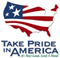Publications— Scientific Investigations Reports

In cooperation with the Pennsylvania Department of Environmental Protection

# Low-Flow, Base-Flow, and Mean-Flow Regression Equations for Pennsylvania Streams

U.S. Geological Survey Scientific Investigations Report 2006-5130

By Marla H. Stuckey

View the full report in PDF 3.2 MB

## ABSTRACT

Low-flow, base-flow, and mean-flow characteristics are an important part of assessing water resources in a watershed. These streamflow characteristics can be used by watershed planners and regulators to determine water availability, water-use allocations, assimilative capacities of streams, and aquatic-habitat needs. Streamflow characteristics are commonly predicted by use of regression equations when a nearby streamflow-gaging station is not available.

Regression equations for predicting low-flow, base-flow, and mean-flow characteristics for Pennsylvania streams were developed from data collected at 293 continuous- and partial-record streamflow-gaging stations with flow unaffected by upstream regulation, diversion, or mining. Continuous-record stations used in the regression analysis had 9 years or more of data, and partial-record stations used had seven or more measurements collected during base-flow conditions. The state was divided into five low-flow regions and regional regression equations were developed for the 7-day, 10-year; 7-day, 2-year; 30-day, 10-year; 30-day, 2-year; and 90-day, 10-year low flows using generalized least-squares regression. Statewide regression equations were developed for the 10-year, 25-year, and 50-year base flows using generalized least-squares regression. Statewide regression equations were developed for harmonic mean and mean annual flow using weighted least-squares regression.

Basin characteristics found to be significant explanatory variables at the 95-percent confidence level for one or more regression equations were drainage area, basin slope, thickness of soil, stream density, mean annual precipitation, mean elevation, and the percentage of glaciation, carbonate bedrock, forested area, and urban area within a basin. Standard errors of prediction ranged from 33 to 66 percent for the n-day, T-year low flows; 21 to 23 percent for the base flows; and 12 to 38 percent for the mean annual flow and harmonic mean, respectively. The regression equations are not valid in watersheds with upstream regulation, diversions, or mining activities. Watersheds with karst features need close examination as to the applicability of the regression-equation results.

Abstract
Introduction
Purpose and Scope
Previous Investigations
Physiography and Drainage
Development of Regression Equations
Streamflow-Gaging Stations
Basin Characteristics
Regression Techniques
Low-Flow Regression Equations
Base-Flow Regression Equations
Mean-Flow Regression Equations
Limitations of Regression Equations
Summary
Acknowledgments
References Cited
Appendix 1. Streamflow-gaging stations used in development of low-flow, base-flow, and mean-flow regression
equations for Pennsylvania streams.
Appendix 2. Partial-record streamflow-gaging-station measurements and correlation results
Appendix 3. Basin characteristics for streamflow-gaging stations used in the development of low-flow, base-flow,
and mean-flow regression equations for Pennsylvania streams.
Appendix 4. Low-flow characteristics computed from streamflow-gaging-station data and regression equations for
stations used in analysis
Appendix 5. Base-flow and mean-flow characteristics computed from streamflow-gaging-station data and regression
equations for stations used in analysis

View the full report in PDF 3.2 MB

 AccessibilityFOIAPrivacyPolicies and Notices U.S. Department of the Interior, U.S. Geological Survey Persistent URL: Page Contact Information: Contact USGS Last modified: Thursday, December 01 2016, 07:08:54 PM